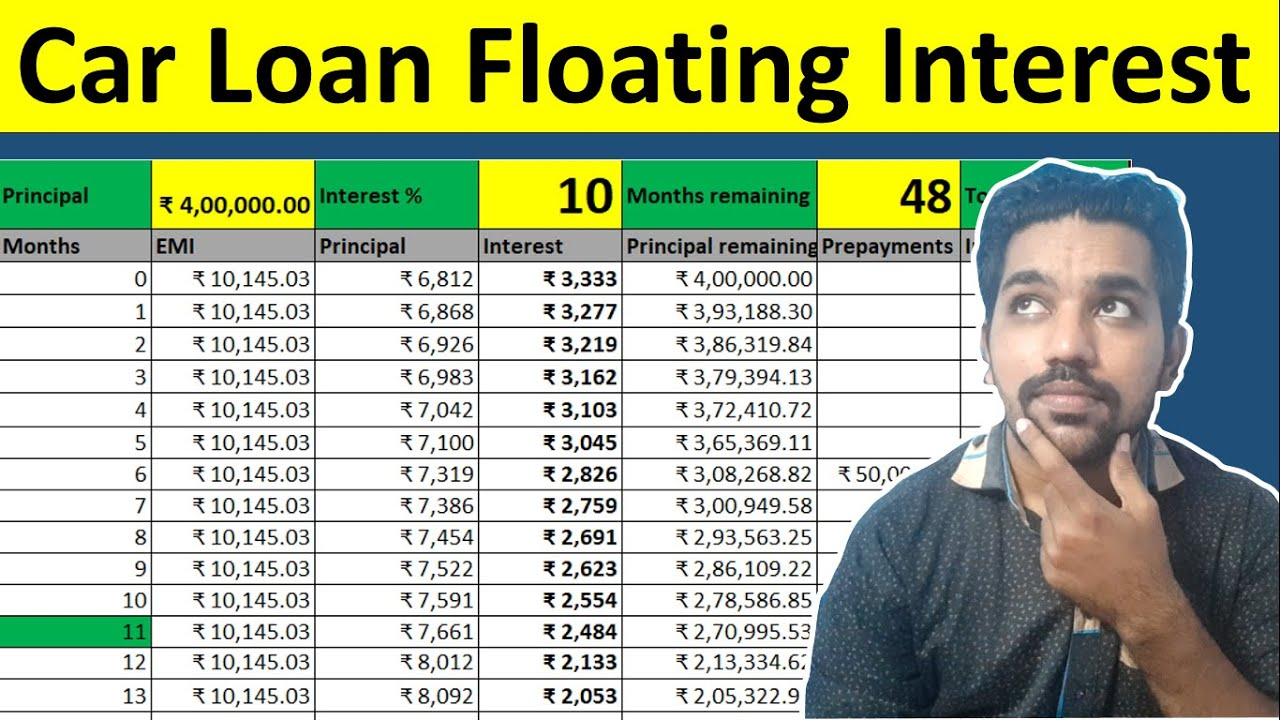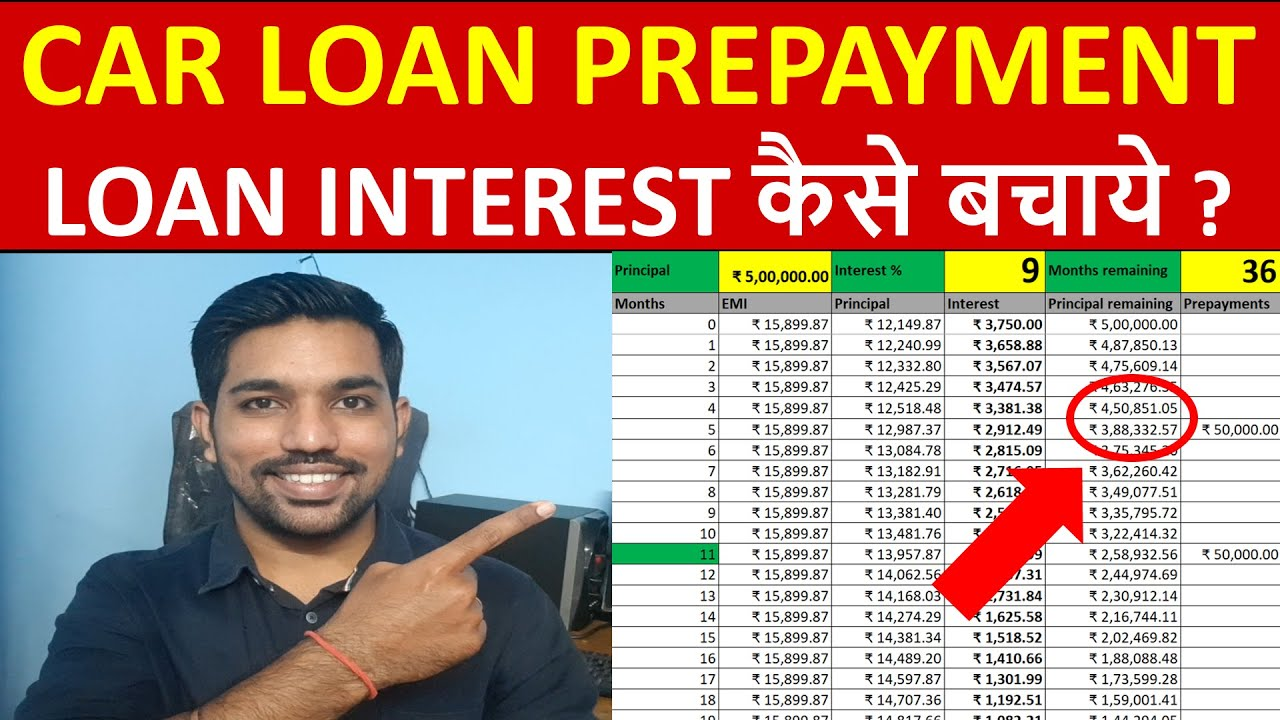# Car Loan Floating Interest Rate Calculation in ExcelWhen you buy a new car on loan, you get 2 types of interest rates on car loan – Fixed and floating interest rates. While fixed interest rate car loan have fixed rate of interest throughout your loan tenure, floating interest rate is where the rate of interest is updated periodically, it can go high or low based on certain economy conditions. We’ll see how floating interest rate car loan can increase your loan tenure when interest rate is increased with calculations in excel.

In Car Loan Floating Interest Rate, when the interest rate increases, your loan tenure increases by default and when interest rate decreases, loan tenure also decreases. Due to the increase in interest rate, the interest amount increases due to which either EMI amount or tenure must increase. So by default, tenure increase is done instead of EMI amount increase. That’s the reason your loan tenure changes suddenly when you see your loan statement after a long time.

You can find the Car Loan Floating Interest Rate excel calculator at the end of this article.

## Car Loan Floating Interest Rate Calculation VideoWatch more Excel Videos Here

As seen in above video, loan tenure increases when interest rate increases in your car loan.

You can make Car Loan Prepayments to save loan interest which we are going to see below.

Use below Car Loan EMI Calculator to check your loan EMI:

## What is floating interest rate on car loan?

• Floating interest rate in car loan changes the interest rate in your loan periodically
• It depends on the economic conditions and when RBI updates repo rate
• Floating interest rate are 1% to 2.5% lower compared to fixed interest rate car loans
• Since fixed interest rates cannot be changed during the loan tenure, they are kept higher compared to floating rates
• As compared to fixed interest rate car loan, you will notice loan tenure changes in floating rate car loans
• In fixed interest rate car loan, the tenure and EMI remains fixed throughout the loan tenure
• Also, in floating rates, you get the option to make car loan prepayments to save loan interest amounts
• There is no penalty while making car loan prepayments in floating rate car loans
• You can close your car loan before time while making prepayments periodically
• Longer the loan tenure means more interest amount you pay in your car loan

## Floating Interest Rate Car Loan Calculation Example

Let us take an example to see how floating rate car loan can impact your loan tenure.

Example:
Loan Amount = Rs. 5 Lakh
Interest Rate = 12%
Tenure = 36 months

Below is the screenshot of calculations when interest rate is fixed:

As seen, you pay total interest amount of Rs. 97,858 throughout the loan tenure for given example.

Below is the screenshot of calculations when interest rate increases by 1% every 6 months:

As seen above, you pay Rs. 1,15,201 as interest amount with floating rate when interest rates are increasing by 1% every 6 months. You pay Rs. 17,342 as extra interest amount compared to the rates that were not increasing previously.

The tenure also increased by 2 months due to this interest rate increase periodically.

## Is SBI car loan interest rate fixed or floating?

You get both types of interest rates in SBI.

By default, car loan in SBI is of floating interest rate, but it is always better to confirm while taking loan.

You also get fixed rate car loan in SBI which will be slightly higher compared to floating rate car loan.

ALSO READ: Home Loan Floating Interest Rate Calculation

## Which car loan is better fixed or floating interest rate?

It depends on your understanding and prediction. Fixed rates are higher compared to floating rate car loan but interest rate in floating type can go higher with time.

If you think that economic conditions will be good and interest rates will not increase in next 3-5 years, you should go for floating rate car loan. But if you see that in near future interest rates will increase and go higher compared to current fixed interest rates in car loan, than you should go for fixed rate car loan.

Below is the comparison of total interest amount you pay on fixed and variable interest rates.

### Interest rate do not change

As seen above, the interest amount you pay in fixed rate car loan is high compared to floating rate car loan.

Let us now see comparison where interest rate changes every 6 months by 1% in floating rate car loan.

### Interest rate changes

As seen above, in the example considered we pay almost same interest amount in both types of loan. But if the tenure is high, floating rate car loan will make us pay more interest amount compared to fixed rate car loan.

## How to Save Car Loan Interest?

Important question is how do you save car loan interest amount you pay?

You can make car loan prepayments to save loan interest amount. Car loan prepayment is a way to deposit some amount against your loan account once you have accumulated and saved the amount.

When you make prepayments, the amount you deposit in your loan account is reduced from your principal outstanding balance of your loan, which decrease the interest portion of your EMI (Equated Monthly Installment) and hence it saves the total interest amount you pay overall.

In above example, if you make loan prepayments of Rs. 50,000 in 6th and 12th month of your loan, you save a total interest amount of Rs. 36,444 and your total interest amount drops back to Rs. 78,439.

Watch below video for more examples.

### Car Loan Prepayment Example VideoWatch more Videos on YouTube Channel

Enter Correct Email ID to receive Excel
If you opt in above, we use this information to send related content## Conclusion

So in floating rate car loan, the interest rate can increase or decrease based on economic conditions and decision of RBI on repo rate.

If your car loan is of floating rate type, you can make car loan prepayments to save loan interest and close loan before time. If you don’t make prepayments than you should expect the increase in loan tenure with time due to increase in interest rate in car loan which is common.

## You can Donate Too!

Found this Helpful? DONATE any amount to see more useful Content. Scan below QR code using any UPI App!

UPI ID: abhilashgupta8149-1@okhdfcbank

Verify that you are “Paying Abhilash Gupta” before making the transaction so that it reaches me. It makes my Day 🙂

Thank you for Donating. Stay Tuned!

## Income Tax Calculator App – FinCalC

For Income Tax Calculation on your mobile device, you can Download my Android App “FinCalC” which I have developed for you to make your income tax calculation easy.

### What you can do with this mobile App?

• Calculate Income Tax for new FY 2023-24 and previous FY 2022-23
• Enter estimated Investments to check income tax with Old and New Tax Regime
• Save income tax details and track regularly
• Know how much to invest more to save income tax
• More calculators including PPF, SIP returns, Savings account interest and lot more